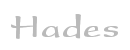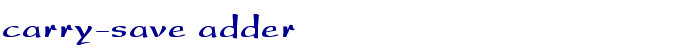TAMS / Java / Hades / applets (print version): contents | previous | next

#### Carry-save adder (6 bits)Description

A Carry-Save Adder is just a set of one-bit fulladders, without any carry-chaining. Therefore, an n-bit CSA receives three n-bit operands, namely A(n-1)..A(0), B(n-1)..B(0), and CIN(n-1)..CIN(0), and generates two n-bit result values, SUM(n-1)..SUM(0) and COUT(n-1)..COUT(0).

The most important application of a carry-save adder is to calculate the partial products in integer multiplication. This allows for architectures, where a tree of carry-save adders (a so called Wallace tree) is used to calculate the partial products very fast. One 'normal' adder is then used to add the last set of carry bits to the last partial products to give the final multiplication result. Usually, a very fast carry-lookahead or carry-select adder is used for this last stage, in order to obtain the optimal performance.

Run the applet | Run the editor (via Webstart)

Impressum | 24.11.06